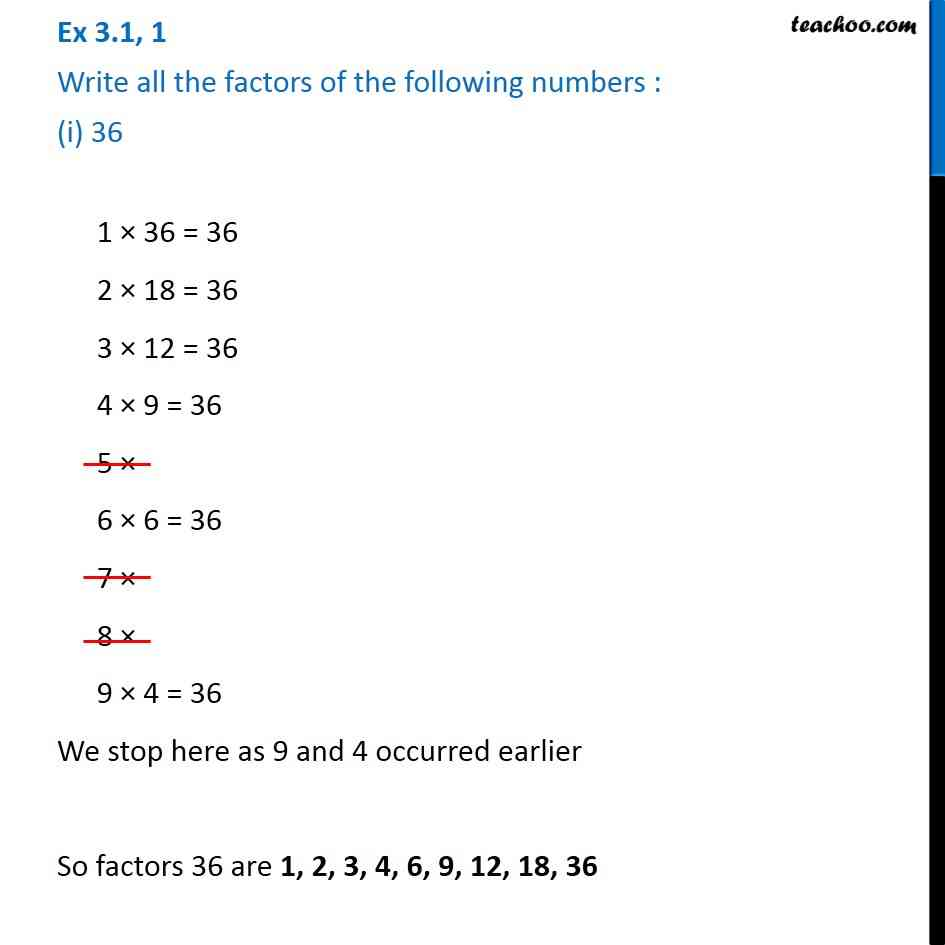Ex 3.1

Chapter 3 Class 6 Playing with Numbers
Serial order wiseLearn in your speed, with individual attention - Teachoo Maths 1-on-1 Class

### Transcript

Ex 3.1, 1 Write all the factors of the following numbers : (i) 36 1 × 36 = 36 2 × 18 = 36 3 × 12 = 36 4 × 9 = 36 5 × 6 × 6 = 36 7 × 8 × 9 × 4 = 36 We stop here as 9 and 4 occurred earlier So factors 36 are 1, 2, 3, 4, 6, 9, 12, 18, 36